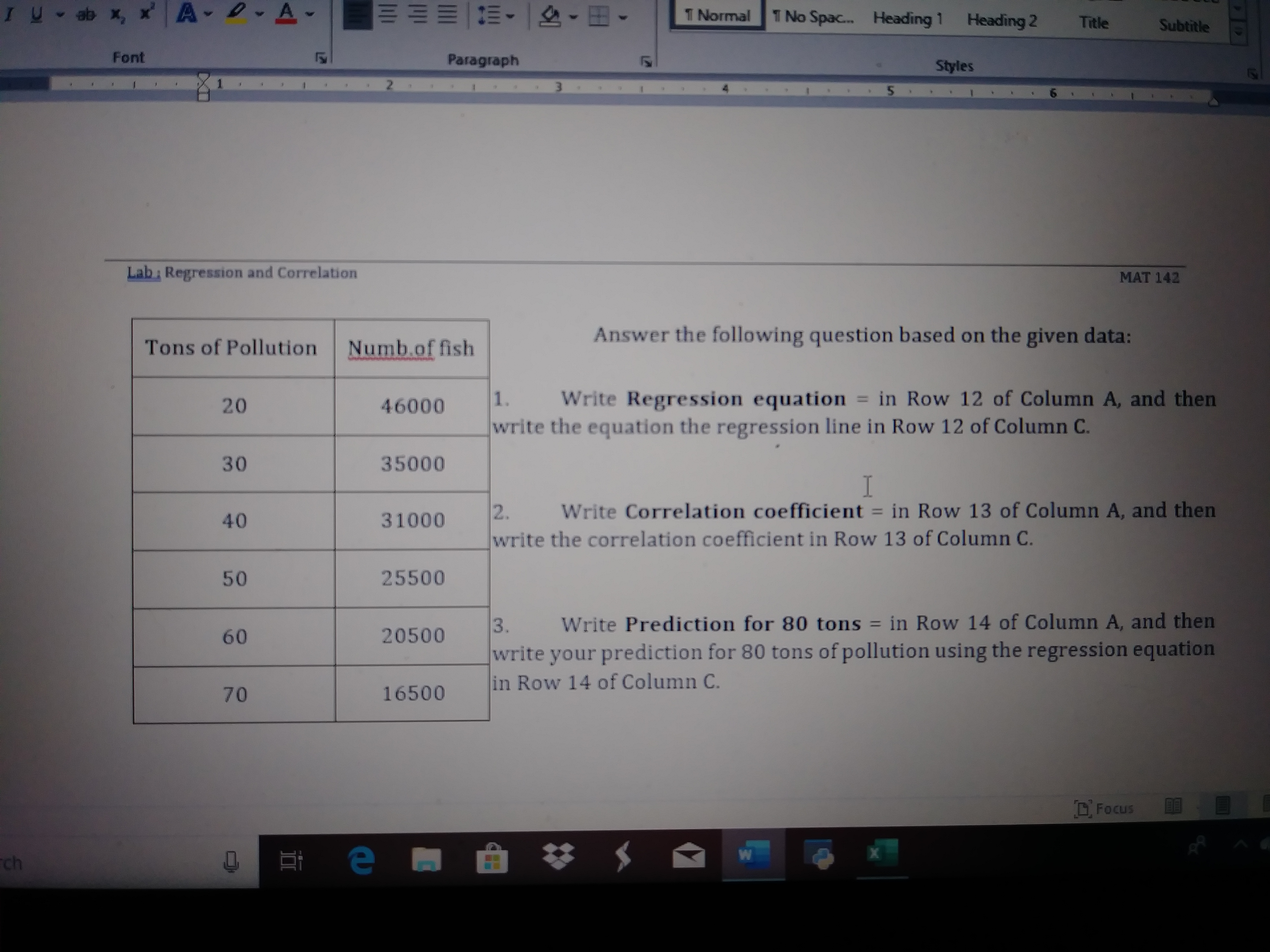# D- A-IU- ab x, x A-T Normal1 No Spac.Heading 1Heading 2TitleSubtitleFontParagraphStyles2.Lab Regression and CorrelationMAT 142Answer the following question based on the given data:Tons of PollutionNumb.of fishWrite Regression equation = in Row 12 of Column A, and then1.%3D2046000write the equation the regression line in Row 12 of Column C.3035000Write Correlation coefficient = in Row 13 of Column A, and then2.write the correlation coefficient in Row 13 of Column C.%3D40310002550050Write Prediction for 80 tons = in Row 14 of Column A, and then3.write your prediction for 80 tons of pollution using the regression equationin Row 14 of Column C.%3D20500601650070D. Focusch

Question
6 views

Question 2help_outlineImage TranscriptioncloseD- A- IU- ab x, x A- T Normal 1 No Spac. Heading 1 Heading 2 Title Subtitle Font Paragraph Styles 2. Lab Regression and Correlation MAT 142 Answer the following question based on the given data: Tons of Pollution Numb.of fish Write Regression equation = in Row 12 of Column A, and then 1. %3D 20 46000 write the equation the regression line in Row 12 of Column C. 30 35000 Write Correlation coefficient = in Row 13 of Column A, and then 2. write the correlation coefficient in Row 13 of Column C. %3D 40 31000 25500 50 Write Prediction for 80 tons = in Row 14 of Column A, and then 3. write your prediction for 80 tons of pollution using the regression equation in Row 14 of Column C. %3D 20500 60 16500 70 D. Focus ch fullscreen
check_circle

Step 1

Given list of data of Tons of pollution and Number of fish.
The predictor variable or independent variable = x = Tons of pollution
The res...

### Want to see the full answer?

See Solution

#### Want to see this answer and more?

Solutions are written by subject experts who are available 24/7. Questions are typically answered within 1 hour.*

See Solution
*Response times may vary by subject and question.
Tagged in

### Data